Unit Conversions (Part 1):

by on June 22nd, 2018Many GMAT Quant problems can easily turn into black holes of tedious and time-consuming calculations. Unit conversions are good examples of this: the GMAT will sometimes test your ability to convert between kilograms and milligrams, or miles and kilometers, or miles per hour and feet per second, and the calculations can get real ugly, real quick. But the good news is that with the right approach, you can usually avoid most—if not all—of the annoying math.

So let’s try one from GMAT Prep. Set a timer for 2 minutes and give it a go:

The main ingredient in a certain prescription drug capsule costs \$500.00 per kilogram. If each capsule contains 600 milligrams of the ingredient, what is the cost of the ingredient in a capsule? (1 kilogram = 106 milligrams)

a) \$0.30
b) \$0.83
c) \$1.20
d) \$3.00
e) \$3.33

In this problem, we’re essentially being asked to scale down an existing ratio of dollars per kilogram to a much smaller quantity of dollars per 600 milligrams. They give us the conversion between kilograms and milligrams (1 kg = 106 mg), but if you already know the basics of the metric system, I think it’s easier to think of it as 1 kilogram = 1000 grams, and 1 gram = 1000 milligrams.

Here’s how I approach every unit conversion problem: I set up the given units as a ratio on the left side of my paper, and I set up my ‘goal’ units as a ratio on the right side of my paper. Then I find a way to connect the two.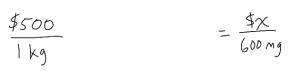On the left, our given units are \$500 per 1 kilogram.

On the right, our goal units are \$x per 600 milligrams.

What that means is that we need to change the weight from kilograms to milligrams, and then we can figure out how many dollars per 600 milligrams. I currently have kilograms in the denominator, but I want to change that to milligrams in the denominator.

To convert from kilograms to milligrams, I’m going to use baby steps—first I’ll convert from kilograms to grams, then convert from grams to milligrams. That might strike some people as inefficient, and maybe it is, but in my opinion, it’s safer, because I’m less likely to screw it up by misplacing a decimal or something.

The key is that in order to convert from one unit to the next, I have to cancel out the units I don’t want in the denominator by matching them with the same units in the numerator (or vice versa). In other words, to convert from dollars per kilogram to dollars per gram, I multiply the given ratio \$500/kg. by 1kg/1000g (which is really just a form of the fraction 1, since 1 kg = 1000 g).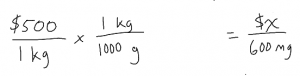Repeat this process to convert from grams to milligrams (1 g = 1000 mg).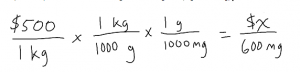Then the kilograms in the denominator (bottom) of the first ratio will cancel out with the kilograms in the numerator (top) of the second ratio, and the grams in the denominator of the second ratio will cancel out with the grams in the numerator of the third ratio.

Cancel out kg with kg and g with g, and you get the ratio \$500 per (1,000 * 1,000) mg.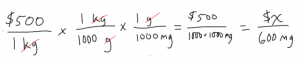Reduce 500/1000 to 1/2: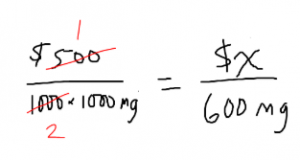and it’s now \$1 per 2,000 mg: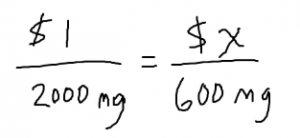Now our milligrams are in the right place: This ingredient costs \$1 per 2000 mg. And hopefully you can see that 2000 mg is close enough to our goal of 600 mg that we can start to do a test of reasonableness to figure out how much 600 mg is worth.

If \$1 buys 2,000 mg, then we already know the answer can’t be C, D, or E since those are all more than \$1, and we only need 600 milligrams’ worth.

For 50 cents we can get 1,000 mg, and half of that (25 cents) would be 500 mg. Since we need 600 mg (a little bit more than 500 mg), the right answer must be a little bit more than 25 cents. Answer A, 30 cents, must be the right answer.

Alternatively, finishing the algebra to solve for x would also get you to the answer. Isolate x by multiplying both sides by 600 mg: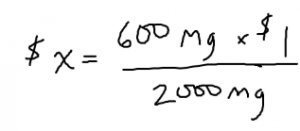Now the milligrams cancel out, and you can reduce 600/2000 to 6/20 and then to 3/10: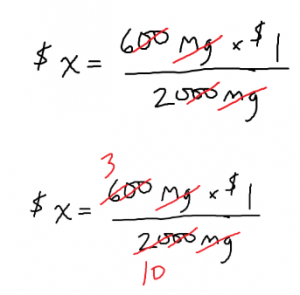So x = 3/10 of a dollar = \$0.30 aka 30 cents.

In Part 2, we’ll learn how to tackle converting units in the Imperial system!